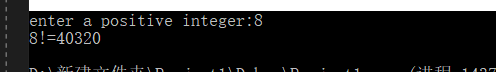# 函数相关

## 三. 函数申明和定义(自定义函数)

	int Add(int number1,int number2);//函数申明

//函数定义

}


## 四. 函数传参

（有一些说有 全局变量传递。 全局变量的优点是效率高，但它对多线程的支持不好，如果两个进程同时调用同一个函数，而通过全局变量进行传递参数，该函数就不能总是得到想要的结果。了解）

• 传值

值传递是单向传递。在函数执行过程中开辟新的空间放传过来的值，相当于复制。下面是一个经典的例子感受一下值传递。

  	#include<iostream>
using namespace std;

void swap(int number1, int number2) {//实现数字交换
int t = number1;
number1 = number2;
number2 = t;
}

int main() {
int x = 10, y = 3;
cout << "x=" << x << "    y=" << y << endl;//打印交换前
swap(x,y);
cout << "x=" << x << "    y=" << y << endl;//打印交换后
return 0;
}


x，y并没有实现交换。因为只是把值传递过去，单向传递，原本的x，y没有被改变。

• 传地址

地址传递与值传递的不同在于，它把实参的存储地址传送给形参，使得形参指针和实参指针指向同一块地址。因此，被调用函数中对形参指针所指向的地址中内容的任何改变都会影响到实参。

  #include<iostream>
using namespace std;

void swap(int *number1, int *number2) {//实现数字交换
int t = *number1;
*number1 = *number2;//因为是地址，所以要用*取值符号
*number2 = t;
}

int main() {
int x = 10, y = 3;
cout << "x=" << x << "    y=" << y << endl;//打印交换前
swap(&x,&y);//注意！ 要传x，y的地址
cout << "x=" << x << "    y=" << y << endl;//打印交换后
return 0;
}


• 传引用

同样的例子，在swap函数参数前加上&，就是给x，y取了一个别名，number1和x代表同一个数。

  	#include<iostream>
using namespace std;

void swap(int &number1, int &number2) {//实现数字交换
int t = number1;
number1 = number2;
number2 = t;
}

int main() {
int x = 10, y = 3;
cout << "x=" << x << "    y=" << y << endl;//打印交换前
swap(x,y);
cout << "x=" << x << "    y=" << y << endl;//打印交换后
return 0;
}


#### 传引用和传地址的异同：

• 相同点：

传引用和传地址，原理上都是将参数变量的地址传递给被调函数。所以在函数内部修改参数的值时，均可返回修改之后的结果给调用者。

• 不同点：

1.引用一定会指向一个对象，而指针可能为空（NULL）； 传引用时，系统对传过来的参数不会有任何额外开销，直接使用原始变量的内存空间。

2.传引用时,函数参数需要写做T&a； 调用函数时直接传递对象本身；在函数内赋值的时候，直接对a赋值即可。

3.传地址时，函数参数需要写作T * p;调用函数时需要传入对象地址； 赋值时需要对 * p赋值。

## 五.重载函数

• ！！注意：

1.编译器不以形参名或者返回值来区分重载函数。

2.不要将功能不同的函数定义为重载函数，以免出现对调用结果的误解，混淆。

  	int add(int x,int y){return x+y;}
float add (float x, float y){ return x-y;}


3.在使用有默认参值时重载函数时，防止二义性。

• 为什么重载函数

1.重载的最直接作用是方便了程序员可以根据不同的参数个数，顺序，类型，自动匹配方法，减少写过多函数名或方法名的重复步骤。方便记忆，便于使用。

2.函数重载用于定义功能相似的同名函数，提高函数的易用性。

	#include<iostream>
using namespace std;

int sumOfSquare(int a,int b) {
return a * a + b * b;
}

double sumOfSquare(double a, double b) { // 重载函数形参类型不同
return a * a + b * b;
}

int main() {
int m, n;//int 型测试
cout << "enter two interget:";
cin >> m >> n;
cout << "their sum of square :" << sumOfSquare(m,n) << endl;

double x, y;//double 测试
cout << "enter two real number:";
cin >> x >> y;
cout << "their sum of square :" << sumOfSquare(x, y) << endl;

return 0;
}


## 六. 递归函数

	#include<iostream>
using namespace std;

unsigned fac(unsigned n) {
unsigned f;
if (n == 0) {
f = 1;   //！要有最后的判定条件
}
else {
f = fac(n - 1) * n; //直接调用自身
}
return f;
}

int main() {
unsigned n;
cout << "enter a positive integer:";
cin >> n;
unsigned y = fac(n);
cout << n << "!=" << y << endl;
return 0;
}## 结语

posted on 2019-09-15 15:13  SailorMoon-z  阅读(536)  评论(0编辑  收藏  举报